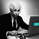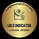62433瀏覽
62433
CM_Ultimate_MA_MTF_V2 - Added Tilson T3

Defaults to Current Timeframe on Chart.
Ability to Plot 2nd Moving Average.
Ability to set Moving Averages to Custom Chart TimeFrame. Example Daily Ma on 60 Minute chart. Many Different Options from Weekly to 1 Minute.
Ability to Plot Cross where Moving Averages Cross (If using 2nd Moving Average).
Ability to Plot Highlight Bars when Price Crosses 1st Moving Average, or 2nd MA.

Moving Averages Supported in Inputs Tab
SMA - Simple Moving Average
EMA - Exponential Moving Average
WMA - Weighted Moving Average
HullMA - Hull Moving Average
VWMA - Volume Weighted Moving Average
RMA - Moving Average used in RSI - Similar to EMA
TEMA - Triple Exponential Moving Average
Tilson T3 - Tilson T3 Moving Average
```//Created by user ChrisMoody 4-24-2014...Updated 7/28/2014 added Tilson T3
//Modified on 5-5-14 for 4apprentice08 with Optional BarColor based on Price  Crossing MA #1, or #2
//Modified on 7-25-2014 to Add in Tilson T3
//Plots The Majority of Moving Averages
//Defaults to Current Chart Time Frame --- But Can Be Changed to Higher Or Lower Time Frames
//2nd MA Capability with Show Crosses Feature
study(title="CM_Ultimate_MA_MTF_V2", shorttitle="CM_Ultimate_MA_MTF_V2", overlay=true)
//inputs
src = close
useCurrentRes = input(true, title="Use Current Chart Resolution?")
resCustom = input(title="Use Different Timeframe? Uncheck Box Above", type=resolution, defval="D")
len = input(20, title="Moving Average Length - LookBack Period")
//periodT3 = input(defval=7, title="Tilson T3 Period", minval=1)
factorT3 = input(defval=7, title="Tilson T3 Factor - *.10 - so 7 = .7 etc.", minval=0)
atype = input(1,minval=1,maxval=8,title="1=SMA, 2=EMA, 3=WMA, 4=HullMA, 5=VWMA, 6=RMA, 7=TEMA, 8=Tilson T3")
spc=input(false, title="Show Price Crossing 1st Mov Avg - Highlight Bar?")
cc = input(true,title="Change Color Based On Direction?")
smoothe = input(2, minval=1, maxval=10, title="Color Smoothing - Setting 1 = No Smoothing")
doma2 = input(false, title="Optional 2nd Moving Average")
spc2=input(false, title="Show Price Crossing 2nd Mov Avg?")
len2 = input(50, title="Moving Average Length - Optional 2nd MA")
sfactorT3 = input(defval=7, title="Tilson T3 Factor - *.10 - so 7 = .7 etc.", minval=0)
atype2 = input(1,minval=1,maxval=8,title="1=SMA, 2=EMA, 3=WMA, 4=HullMA, 5=VWMA, 6=RMA, 7=TEMA, 8=Tilson T3")
cc2 = input(true,title="Change Color Based On Direction 2nd MA?")
warn = input(false, title="***You Can Turn On The Show Dots Parameter Below Without Plotting 2nd MA to See Crosses***")
warn2 = input(false, title="***If Using Cross Feature W/O Plotting 2ndMA - Make Sure 2ndMA Parameters are Set Correctly***")
sd = input(false, title="Show Dots on Cross of Both MA's")

res = useCurrentRes ? period : resCustom
//hull ma definition
hullma = wma(2*wma(src, len/2)-wma(src, len), round(sqrt(len)))
//TEMA definition
ema1 = ema(src, len)
ema2 = ema(ema1, len)
ema3 = ema(ema2, len)
tema = 3 * (ema1 - ema2) + ema3

//Tilson T3
factor = factorT3 *.10
gd(src, len, factor) => ema(src, len) * (1 + factor) - ema(ema(src, len), len) * factor
t3(src, len, factor) => gd(gd(gd(src, len, factor), len, factor), len, factor)
tilT3 = t3(src, len, factor)

avg = atype == 1 ? sma(src,len) : atype == 2 ? ema(src,len) : atype == 3 ? wma(src,len) : atype == 4 ? hullma : atype == 5 ? vwma(src, len) : atype == 6 ? rma(src,len) : atype == 7 ? 3 * (ema1 - ema2) + ema3 : tilT3
//2nd Ma - hull ma definition
hullma2 = wma(2*wma(src, len2/2)-wma(src, len2), round(sqrt(len2)))
//2nd MA TEMA definition
sema1 = ema(src, len2)
sema2 = ema(sema1, len2)
sema3 = ema(sema2, len2)
stema = 3 * (sema1 - sema2) + sema3

//2nd MA Tilson T3
sfactor = sfactorT3 *.10
sgd(src, len2, sfactor) => ema(src, len2) * (1 + sfactor) - ema(ema(src, len2), len2) * sfactor
st3(src, len2, sfactor) => sgd(sgd(gd(src, len2, sfactor), len2, sfactor), len2, sfactor)
stilT3 = st3(src, len2, sfactor)

avg2 = atype2 == 1 ? sma(src,len2) : atype2 == 2 ? ema(src,len2) : atype2 == 3 ? wma(src,len2) : atype2 == 4 ? hullma2 : atype2 == 5 ? vwma(src, len2) : atype2 == 6 ? rma(src,len2) : atype2 == 7 ? 3 * (ema1 - ema2) + ema3 : stilT3

out = avg
out_two = avg2

out1 = security(tickerid, res, out)
out2 = security(tickerid, res, out_two)

//Formula for Price Crossing Moving Average #1
cr_up = open < out1 and close > out1
cr_Down = open > out1 and close < out1
//Formula for Price Crossing Moving Average #2
cr_up2 = open < out2 and close > out2
cr_Down2 = open > out2 and close < out2
//barcolor Criteria for Price Crossing Moving Average #1
iscrossUp() => cr_up
iscrossDown() => cr_Down
//barcolor Criteria for Price Crossing Moving Average #2
iscrossUp2() => cr_up2
iscrossDown2() => cr_Down2

ma_up = out1 >= out1[smoothe]
ma_down = out1 < out1[smoothe]

col = cc ? ma_up ? lime : ma_down ? red : aqua : aqua
col2 = cc2 ? ma_up ? lime : ma_down ? red : aqua : white

circleYPosition = out2

plot(out1, title="Multi-Timeframe Moving Avg", style=line, linewidth=4, color = col)
plot(doma2 and out2 ? out2 : na, title="2nd Multi-TimeFrame Moving Average", style=circles, linewidth=4, color=col2)
plot(sd and cross(out1, out2) ? circleYPosition : na,style=cross, linewidth=15, color=aqua)
//barcolor Plot for Price Crossing Moving Average #1
barcolor(spc and iscrossUp() ? (iscrossUp() ? yellow : na) : na)
barcolor(spc and iscrossDown() ? (iscrossDown() ? yellow : na) : na)
//barcolor Plot for Price Crossing Moving Average #2
barcolor(spc2 and iscrossUp2() ? (iscrossUp2() ? yellow : na) : na)
barcolor(spc2 and iscrossDown2() ? (iscrossDown2() ? yellow : na) : na)```@ChrisMoody hell of an indicator! Well done sir. Popular demand appears to be for the alert/V3, in that the alerts are triggered per change in colour as opposed to each bar. Would you have had time to share this with us? ThanksGreat one ,ultimately great one ,i personally use this for my trades with this auto scripts